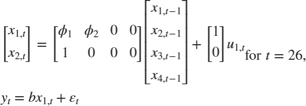# Estimate Time-Varying Diffuse State-Space Model

This example shows how to create and estimate a diffuse state-space model containing time-varying parameters.

Suppose that an AR(2) and an MA(1) model comprise a latent process. There are 50 periods, and the MA(1) process drops out of the model for the final 25 periods. Consequently, the state equation for the first 25 periods isFor the last 25 periods, the state equation iswhereandare Gaussian with mean 0 and standard deviation 1.

Generate a random series of 50 observations fromand, assuming that the series starts at 1.5 and 1, respectively.

T = 50; ARMdl = arima('AR',{0.7,-0.2},'Constant',0,'Variance',1); MAMdl = arima('MA',0.6,'Constant',0,'Variance',1); x0 = [1.5 1; 1.5 1]; rng(1); x = [simulate(ARMdl,T,'Y0',x0(:,1)),... [simulate(MAMdl,T/2,'Y0',x0(:,2));nan(T/2,1)]]; 

The last 25 values for the simulated MA(1) data are missing.

The latent processes are measured usingfor the first 25 periods, andfor the last 25 periods.is Gaussian with mean 0 and standard deviation 1.

Generate observations using the random, latent state process (x) and the observation equation.

y = 2*sum(x','omitnan')' + randn(T,1); 

Together, the latent process and observation equations make up a state-space model. If the coefficients are unknown parameters, the state-space model isWrite a function that specifies how the parameters in params map to the state-space model matrices, the initial state values, and the type of state.

 % Copyright 2015 The MathWorks, Inc. function [A,B,C,D,Mean0,Cov0,StateType] = diffuseAR2MAParamMap(params,T) %diffuseAR2MAParamMap Time-variant diffuse state-space model parameter %mapping function % % This function maps the vector params to the state-space matrices (A, B, % C, and D) and the type of state (StateType). From periods 1 to T/2, the % state model is an AR(2) and an MA(1) model, and the observation model is % the sum of the two states. From periods T/2 + 1 to T, the state model is % just the AR(2) model. The AR(2) model is diffuse. A1 = {[params(1) params(2) 0 0; 1 0 0 0; 0 0 0 params(3); 0 0 0 0]}; B1 = {[1 0; 0 0; 0 1; 0 1]}; C1 = {params(4)*[1 0 1 0]}; Mean0 = []; Cov0 = []; StateType = [2 2 0 0]; A2 = {[params(1) params(2) 0 0; 1 0 0 0]}; B2 = {[1; 0]}; A3 = {[params(1) params(2); 1 0]}; B3 = {[1; 0]}; C3 = {params(5)*[1 0]}; A = [repmat(A1,T/2,1);A2;repmat(A3,(T-2)/2,1)]; B = [repmat(B1,T/2,1);B2;repmat(B3,(T-2)/2,1)]; C = [repmat(C1,T/2,1);repmat(C3,T/2,1)]; D = 1; end 

Save this code in a file named diffuseAR2MAParamMap on your MATLAB® path.

Create the state-space model by passing the function diffuseAR2MAParamMap as a function handle to dssm.

Mdl = dssm(@(params)diffuseAR2MAParamMap(params,T)); 

dssm implicitly defines the diffuse state-space model. Usually, you cannot verify diffuse state-space models that are implicitly created.

To estimate the parameters, pass the observed responses (y) to estimate . Specify positive initial values for the unknown parameters.

params0 = 0.1*ones(5,1); EstMdl = estimate(Mdl,y,params0) 
Method: Maximum likelihood (fminunc) Effective Sample size: 48 Logarithmic likelihood: -110.313 Akaike info criterion: 230.626 Bayesian info criterion: 240.186 | Coeff Std Err t Stat Prob --------------------------------------------------- c(1) | 0.44041 0.27687 1.59069 0.11168 c(2) | 0.03949 0.29585 0.13349 0.89380 c(3) | 0.78364 1.49222 0.52515 0.59948 c(4) | 1.64260 0.66736 2.46134 0.01384 c(5) | 1.90409 0.49374 3.85648 0.00012 | | Final State Std Dev t Stat Prob x(1) | -0.81932 0.46706 -1.75420 0.07940 x(2) | -0.29909 0.45939 -0.65107 0.51500 EstMdl = State-space model type: <a href="matlab: doc dssm">dssm</a> State vector length: Time-varying Observation vector length: 1 State disturbance vector length: Time-varying Observation innovation vector length: 1 Sample size supported by model: 50 State variables: x1, x2,... State disturbances: u1, u2,... Observation series: y1, y2,... Observation innovations: e1, e2,... State equations of period 1, 2, 3,..., 25: x1(t) = (0.44)x1(t-1) + (0.04)x2(t-1) + u1(t) x2(t) = x1(t-1) x3(t) = (0.78)x4(t-1) + u2(t) x4(t) = u2(t) State equations of period 26: x1(t) = (0.44)x1(t-1) + (0.04)x2(t-1) + u1(t) x2(t) = x1(t-1) State equations of period 27, 28, 29,..., 50: x1(t) = (0.44)x1(t-1) + (0.04)x2(t-1) + u1(t) x2(t) = x1(t-1) Observation equation of period 1, 2, 3,..., 25: y1(t) = (1.64)x1(t) + (1.64)x3(t) + e1(t) Observation equation of period 26, 27, 28,..., 50: y1(t) = (1.90)x1(t) + e1(t) Initial state distribution: Initial state means x1 x2 x3 x4 0 0 0 0 Initial state covariance matrix x1 x2 x3 x4 x1 Inf 0 0 0 x2 0 Inf 0 0 x3 0 0 1.61 1 x4 0 0 1 1 State types x1 x2 x3 x4 Diffuse Diffuse Stationary Stationary 

The estimated parameters are within one standard error of their true values, but the standard errors are quite high. Likelihood surfaces of state-space models might contain local maxima. Therefore, try several initial parameter values, or consider using refine.